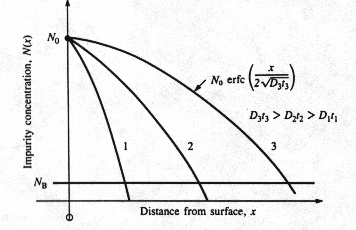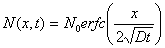# Constant-Source DiffusionFig 2 Constant-source diffusion results in a complementary error function impurity distribution. The surface concentration N0 remains constant and the diffusion moves deeper into the silicon wafer as the Dt product increases. Dt can change as a result of increasing diffusion time, increasing diffusion temperature, or a combination of both. 

A constant-source diffusion or constant surface concentration or solid solubility limited predeposition / diffusion results in a complementary error function impurity distribution. The surface concentration N0 remains constant and the diffusion moves deeper into the silicon wafer as Dt product increases. Dt can change as a result of increasing diffusion time, increasing diffusion temperature, or a combination of both.

Initial Condition:

At time t=0, impurity concentration at depth x and time t, that is, N(x,t) is given by,

N (x, 0) = 0

Boundary Conditions:

At surface we have N(0,t) = N0 and N(¥,t) = 0 for x=¥

The solution of Fick's second law that satisfies the initial and boundary conditions is given byWhere N0 is the surface concentration (atoms/cm2)

D is constant diffusivity in cm2/s

x is the distance in cm

t is the diffusion time in seconds

The table below gives some values of z= x/2(Dt)1/2 and erfc(z)

 z erfc(z) 0 1.0 0.5 0.5 1.0 1.7 1.5 0.35 2.0 0.005 2.5 0.0004 3.0 0.00002 ¥ 0